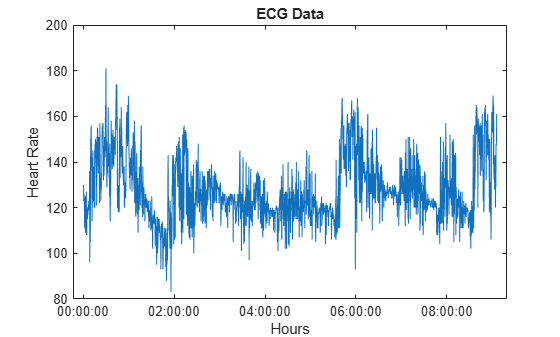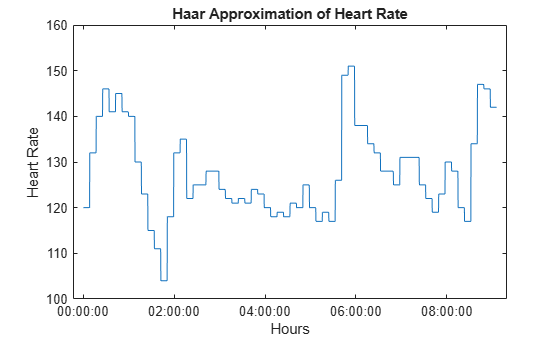Main Content

# haart

Haar 1-D wavelet transform

## Syntax

``````[a,d] = haart(x)``````
``````[a,d] = haart(x,level)``````
``````[a,d] = haart(___,integerflag)``````

## Description

example

``````[a,d] = haart(x)``` performs the 1-D Haar discrete wavelet transform of the even-length vector, `x`. The input `x` can be univariate or multivariate data. If `x` is a matrix, `haart` operates on each column of `x`. If the length of `x` is a power of 2, the Haar transform is obtained down to level `log2(length(x))`. Otherwise, the Haar transform is obtained down to level `floor(log2(length(x)/2))`.```

example

``````[a,d] = haart(x,level)``` obtains the Haar transform down to the specified level.```

example

``````[a,d] = haart(___,integerflag)``` specifies how the Haar transform handles integer-valued data, using any of the previous syntaxes.```

## Examples

collapse all

Obtain the Haar transform down to the default maximum level.

```load wecg; [a,d] = haart(wecg);```

Obtain the Haar transform of a multivariate time series dataset of electricity consumption data down to level 4. The `signals` data is transposed so that each time series is in a column, rather than a row.

```load elec35_nor; signals = signals'; [a,d] = haart(signals,4);```

Obtain the Haar transform and inverse Haar transform of ECG heart rate data. The data is made up of integers only.

Load and plot the ECG data.

```load BabyECGData; plot(times,HR) xlabel('Hours') ylabel('Heart Rate') title('ECG Data')```Obtain the Haar transform. Then, obtain the inverse Haar transform approximated at level 5. The scale for this level is 512 seconds, which is ${2}^{5}$ times the sampling interval (16 seconds).

```[a,d] = haart(HR,'integer'); HaarHR = ihaart(a,d,5,'integer');```

Compare the reconstructed data to the original data.

```figure; plot(times,HaarHR) xlabel('Hours') ylabel('Heart Rate') title('Haar Approximation of Heart Rate')```## Input Arguments

collapse all

Input signal, specified as real-valued vector or matrix. If `x` is a vector, it must be even length. If `x` is a matrix, each column must be even length, and `haart` operates on each column of `x`.

Data Types: `single` | `double`

Maximum level to which to perform the Haar transform, specified as a positive integer.

• If the length of `x` is a power of two, `level` is a positive integer less than or equal to `log2(length(x))`.

• If the length of `x` is even, but not a power of two, `level` is a positive integer less than or equal to `floor(log2(length(x)/2))`.

If `level` is 1, the detail coefficients, `d`, are returned as a vector or matrix, depending on whether the input is a vector or matrix, respectively.

Integer-valued data handling, specified as either `'noninteger'` or `'integer'`. `'noninteger'` does not preserve integer-valued data in the Haar transform, and `'integer'` preserves it. The `'integer'` option applies only if all elements of the input, `x`, are integers. For integer-valued input, `haart` returns integer-valued wavelet coefficients. For both `'noninteger'` and `'integer'`, however, the Haar transform algorithm uses floating-point arithmetic. If `x` is single precision, the Haar transform coefficients are single precision. For all other numeric type, the numeric type of the coefficients is double precision.

## Output Arguments

collapse all

Approximation coefficients at the coarsest level, returned as a scalar, vector, or matrix of coefficients, depending on the level to which the transform is calculated. Approximation, or scaling, coefficients are a lowpass representation of the input. At each level, the approximation coefficients are divided into coarser approximation and detail coefficients.

Data Types: `single` | `double`

Detail coefficients, returned as a scalar, vector, matrix, or cell array. Detail coefficients are generally referred to as wavelet coefficients. The number of detail coefficients depends on the selected level and the length of the input. If `d` is a cell array, the elements of `d` are ordered from finest to coarsest resolution.

Note: Generated C and C++ code always returns the wavelet coefficients `d` in a cell array.

Data Types: `single` | `double`

## See Also

Introduced in R2016b

## SupportGet trial now